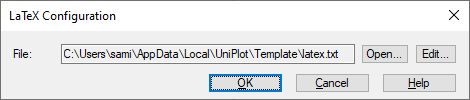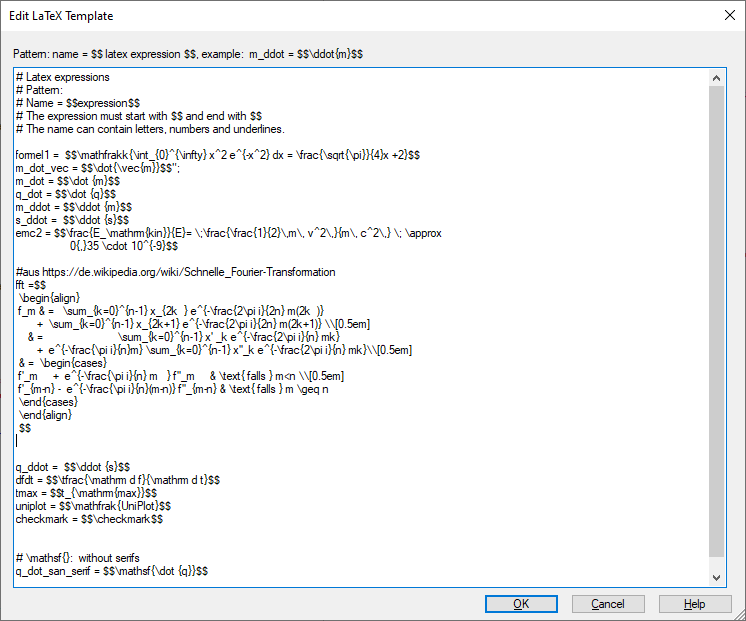# Tools=>LaTeX Formula Configuration¶

(TeX is a typesetting system designed and mostly written by Donald E. Knuth. LaTeX is a typesetting system by Leslie Lamport, using TeX.))

With this command LaTeX formulas can be defined. The LaTeX formulas are stored in a text file. The field function @f{latex} can be used to display the LaTeX formula in a Text object.

See LaTeX <http://en.wikipedia.org/wiki/LaTeX>.File

Is the name of a text file with LaTeX formulas. The default file is located in the template directory of UniPlot. The file name is `latex.txt`. The first time the function is invoked the default file will be copied into the UniPlot user directory.

Open

Opens an Dialogbox to edit the formula text.

Edit

Opens the following simple editor. The file can also be opened with any other text editor:Each LaTeX text formula consists of a name followed by an equal sign, followed by the formula text enclosed in double dollar characters. Example:

```m_dot = \$\$dot{m}\$\$
```

The formula text can be multiline. For multiline formulas, the next line must start with a space. The file can contain any number of formulas. A comments begins with a # sign.

Comment

When closing with OK, a PNG file is created for each LaTeX formula in the UniPlot user directory in the directory `LaTexCache`. The file names are saved in `_g().oLatex`.

To insert the field function into a table cell, double click the cell and then press the button `Insert field function`. In the dialog box, select the @f{latex} field function. Choose a formula from the right list.

id-785188